# SCIENTIFIC NOTATION Scientific Notation is a way to

• Slides: 23SCIENTIFIC NOTATION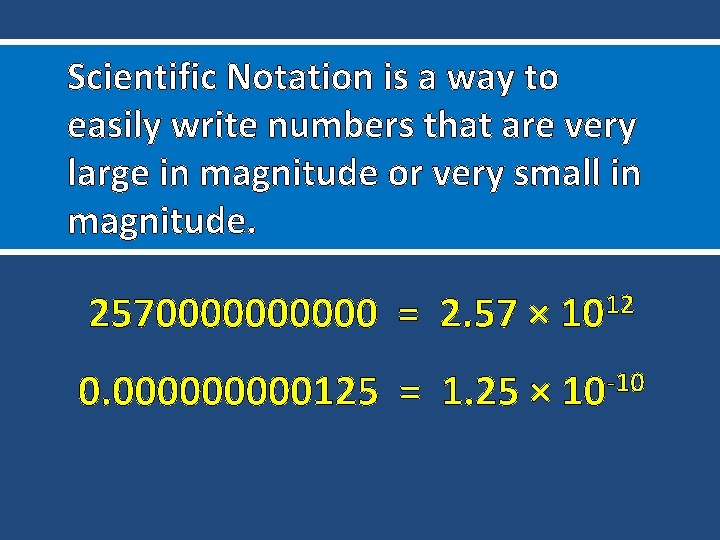Scientific Notation is a way to easily write numbers that are very large in magnitude or very small in magnitude. 25700000 = 2. 57 × 1012 0. 00000125 = 1. 25 × -10 10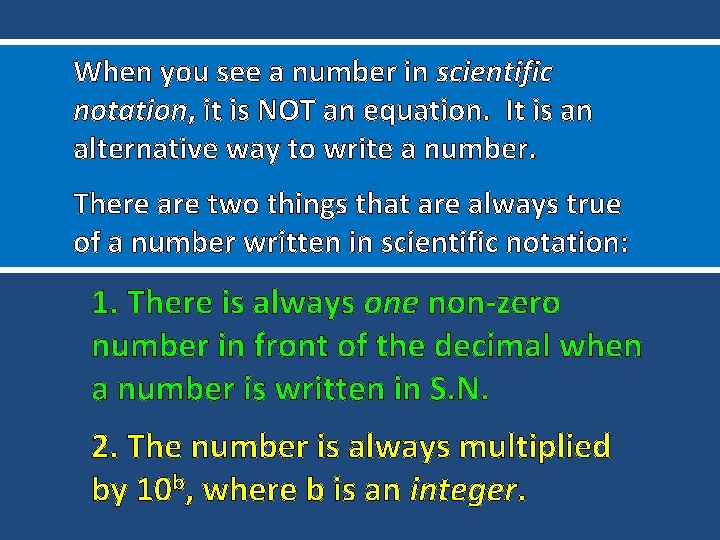When you see a number in scientific notation, it is NOT an equation. It is an alternative way to write a number. There are two things that are always true of a number written in scientific notation: 1. There is always one non-zero number in front of the decimal when a number is written in S. N. 2. The number is always multiplied by 10 b, where b is an integer.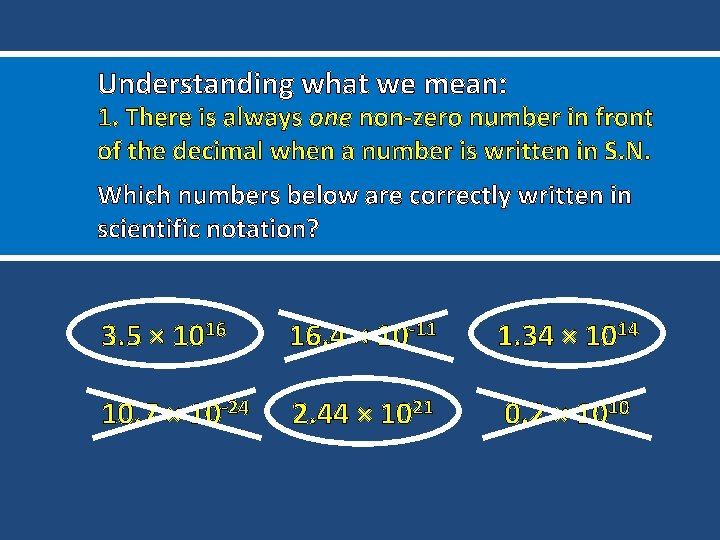Understanding what we mean: 1. There is always one non-zero number in front of the decimal when a number is written in S. N. Which numbers below are correctly written in scientific notation? 3. 5 × 1016 16. 4 × 10 -11 1. 34 × 1014 10. 7 × 10 -24 2. 44 × 1021 0. 2 × 1010Understanding what we mean: 2. The number is always multiplied by 10 b, where b is an integer. Which numbers below are correctly written in scientific notation? 1. 5 × 10 -1. 6 7. 2 × 10 -11 9. 25 × 1014 5. 20 × 10 -21 3. 24 × 102. 5 8. 3 × 101. 25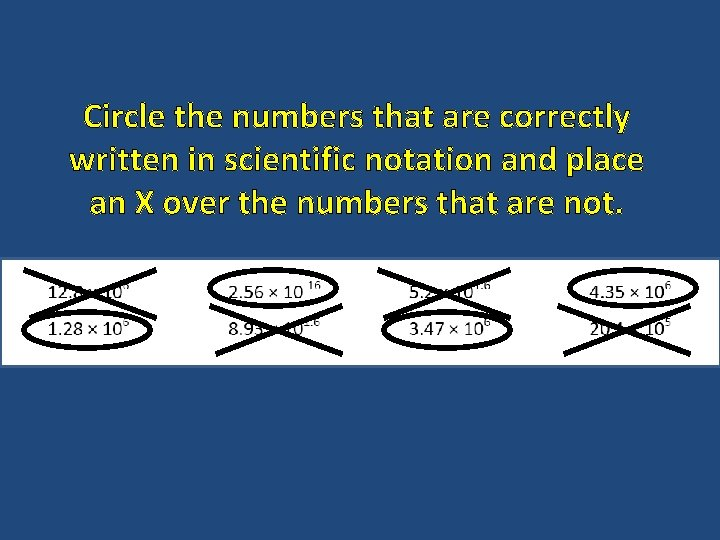Circle the numbers that are correctly written in scientific notation and place an X over the numbers that are not.Understanding when to use positive or negative exponents: Which number written in scientific notation correctly represents the number below? 21800000 2. 18 × 1011 OR 2. 18 × 10 -11 0. 000000145 1. 45 × 1013 OR 1. 45 × 10 -13Which number written in scientific notation correctly represents the number below? 0. 00000287 2. 87 × 1010 OR 2. 87 × 10 -10 96810000000 9. 681 × 1016 OR 9. 681 × 10 -16 62000000 6. 2 × 1013 OR 6. 2 × 10 -13Which number written in scientific notation correctly represents the number below? 0. 00000129 1. 29 × 1010 OR 1. 29 × 10 -10 0. 0000301 3. 01 × 109 OR 3. 01 × 10 -9 1206000000 1. 206 × 1014 OR 1. 206 × 10 -14Describe what a positive and negative exponent means: A positive exponent means that the number is very large in magnitude. Example: 275000000 A negative exponent means that the number is very small in magnitude. Example: 0. 000000275 Understanding this simple concept will keep you from having to memorize whether the decimal should move to the left or the right!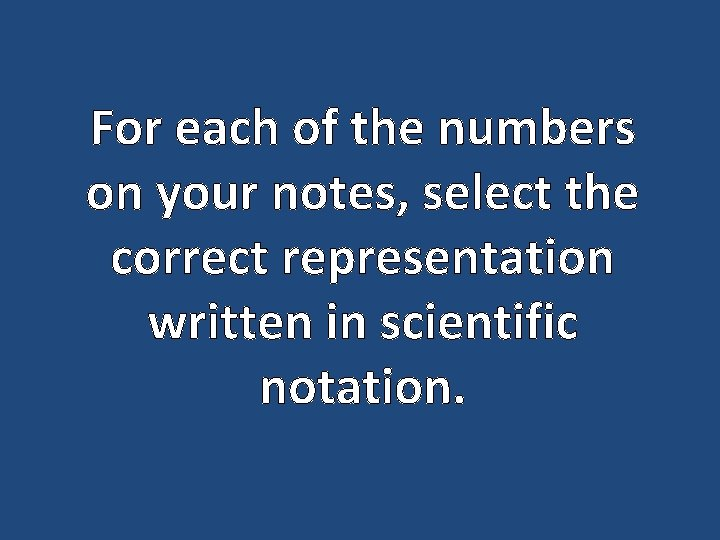For each of the numbers on your notes, select the correct representation written in scientific notation.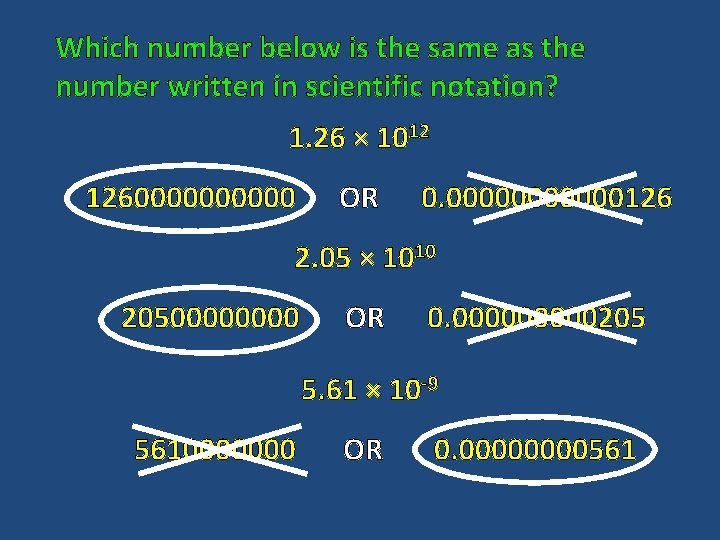Which number below is the same as the number written in scientific notation? 1. 26 × 1012 12600000 OR 0. 000000126 2. 05 × 1010 2050000 OR 0. 00000205 5. 61 × 10 -9 5610000000 OR 0. 0000561Which number below is the same as the number written in scientific notation? 7. 35 × 108 OR 735000000 0. 0000000735 8. 05 × 10 -10 8050000 OR 0. 00000805 1. 3 × 10 -11 1300000 OR 0. 0000013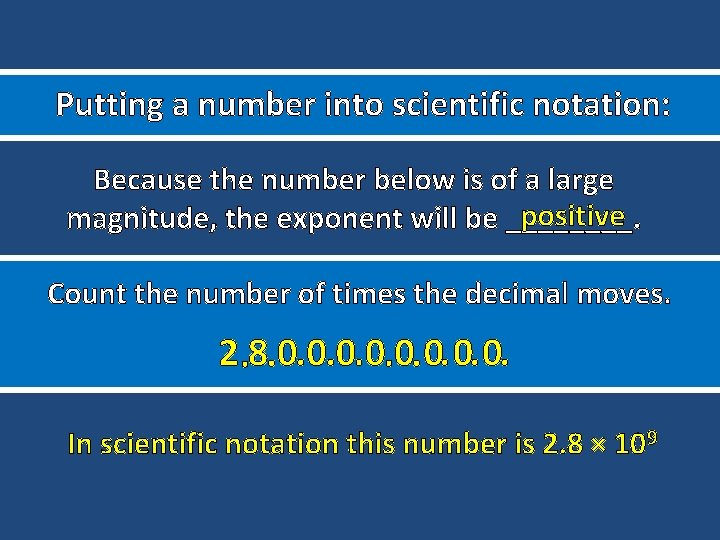Putting a number into scientific notation: Because the number below is of a large positive magnitude, the exponent will be ____. Count the number of times the decimal moves. 2. 8. 0. 0. In scientific notation this number is 2. 8 × 109Putting a number into scientific notation: Because the number below is of a small negative magnitude, the exponent will be ____. Count the number of times the decimal moves. 0. 0. 1. 2 5 In scientific notation this number is 1. 25 × 10 -7Write each of the numbers on your notes page in scientific notation correctly.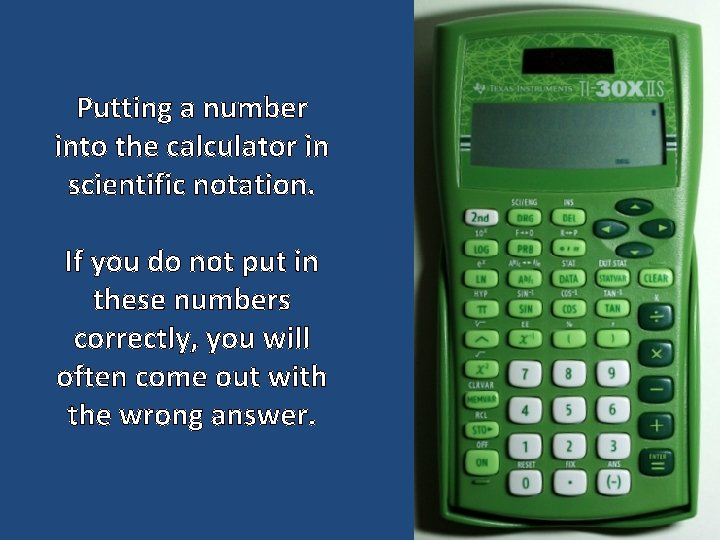Putting a number into the calculator in scientific notation. If you do not put in these numbers correctly, you will often come out with the wrong answer.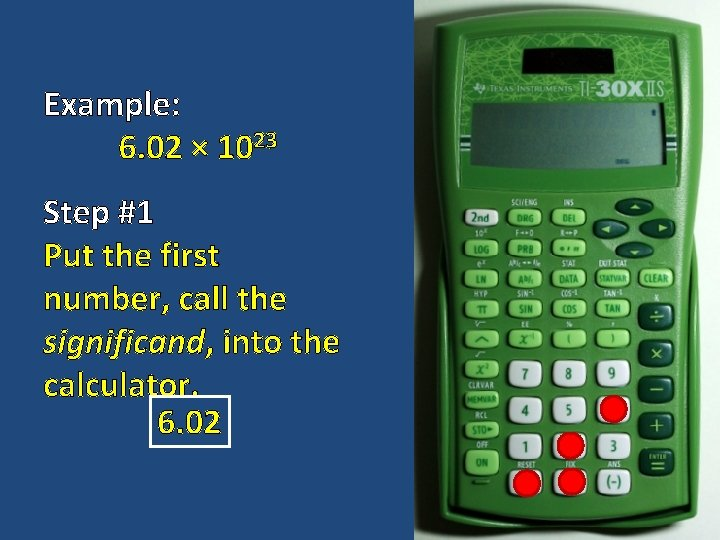Example: 6. 02 × 1023 Step #1 Put the first number, call the significand, into the calculator. 6. 02Example: 6. 02 × 1023 Step #2 Press the key Step #3 Press the keyExample: 6. 02 × 1023 Step #4 Type in the exponent. 23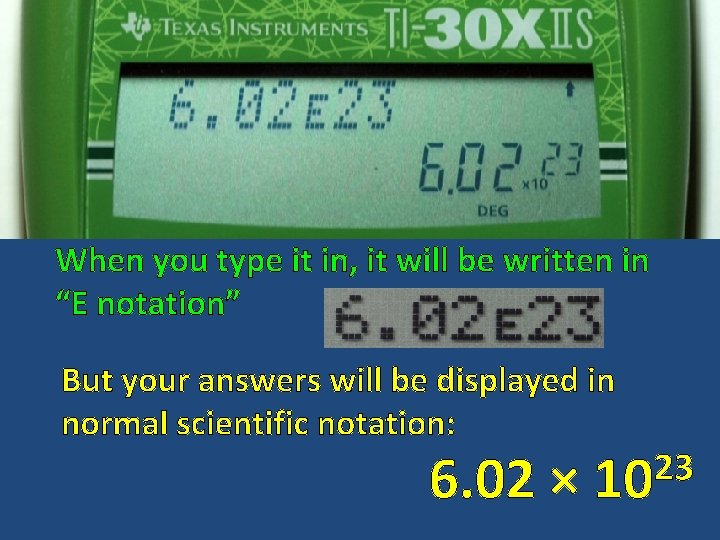When you type it in, it will be written in “E notation” But your answers will be displayed in normal scientific notation: 6. 02 × 23 10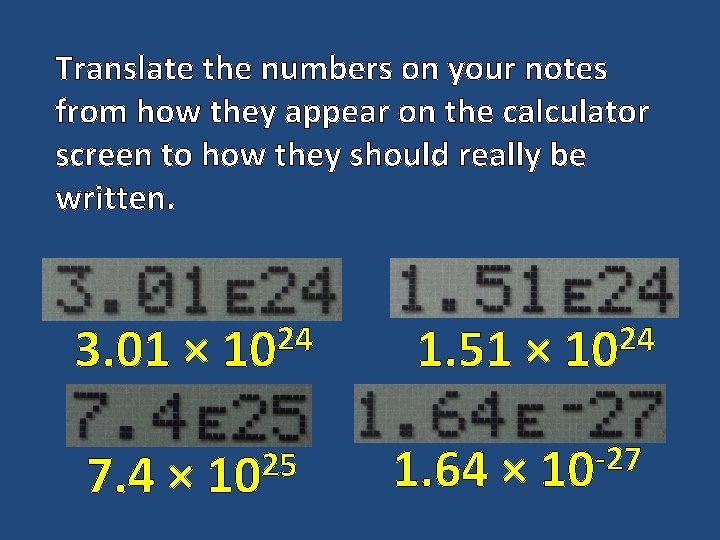Translate the numbers on your notes from how they appear on the calculator screen to how they should really be written. 3. 01 × 7. 4 × 24 10 25 10 1. 51 × 1. 64 × 24 10 -27 10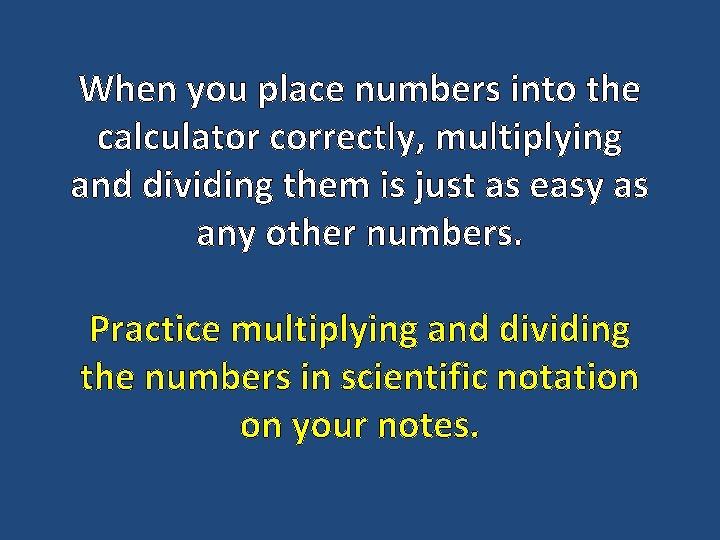When you place numbers into the calculator correctly, multiplying and dividing them is just as easy as any other numbers. Practice multiplying and dividing the numbers in scientific notation on your notes.